# 基于ccs的LMS自适应滤波器设计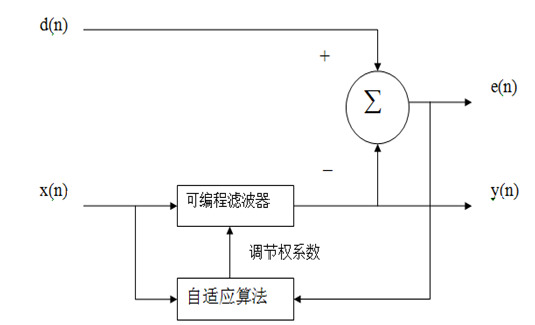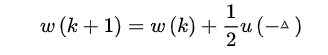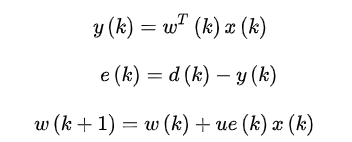LMS自适应算法是一种特殊的梯度估计，不必重复使用数据，也不必对相关矩阵和互相关矩阵进行运算，只需要在每次迭代时利用输入向量和期望响应，结构简单，易于实现。虽然LMS收敛速度较慢，但在解决许多实际中的信号处理问题，LMS算法是仍然是好的选择。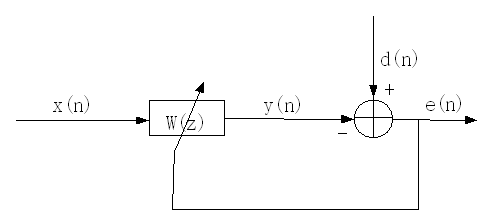1. 初始化各种参量包括自适应滤波器（向量）W (n), 参考信号（向量） X(n)
2. 获取参考信号x(n)和期望信号d(n)
3. 更新参考信号（向量） X(n)
4. 计算滤波信号 y(n) =W (n)X(n)
5. 计算误差信号 e(n)=d(n)-y(n)
6. 更新自适应滤波器 W(n+1)=W(n)+e(n)X(n)
7. 回到第2步进行迭代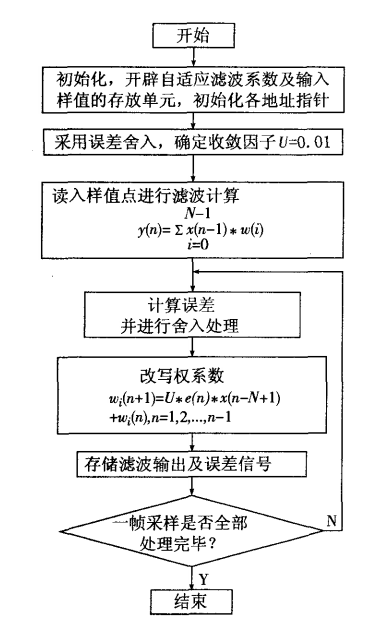四、滤波器C语言代码
#include "math.h"
#include "stdio.h"
int     N=16;                            //滤波器阶数
float   u=0.005;                       //LMS的收敛步长
float   pi=3.1415926;
int     i,j;
float   x;
float   y;
float   d;
float   e;
float   X;
float   W;
void main()
{
for (i=0;i<1024;i++)                //参考信号
{
x[i]=sin((9*i-36)*pi/180);
}

for (i=0;i<1024;i++)                //期望信号
{
d[i]=x[i+N-1];
}

for (i=0;i<N;i++)                  //初始化滤波器参数
{
W[i]=0;
}

for (i=0;i<1024;i++)
{
y[i]=0;
for(j=0;j<N;j++)
{
X[j]=x[i+N-j-1];
}
for (j=0;j<N;j++)
{
y[i]=y[i]+W[j]*X[j];       //计算滤波信号
}
e[i]=d[i]-y[i];
for(j=0;j<N;j++)
{
W[j]=W[j]+2*u*e[i]*X[j];   //更新自适应滤波器
}
}
}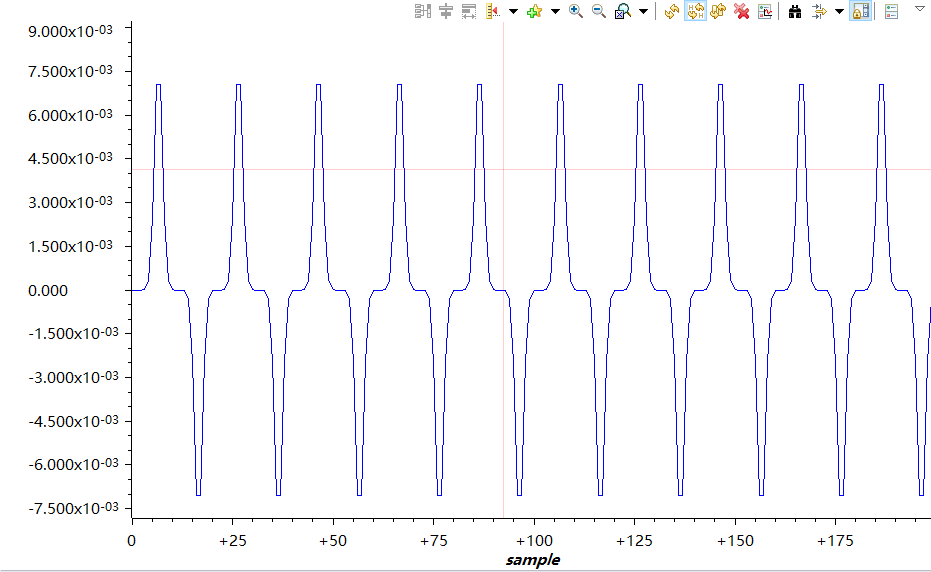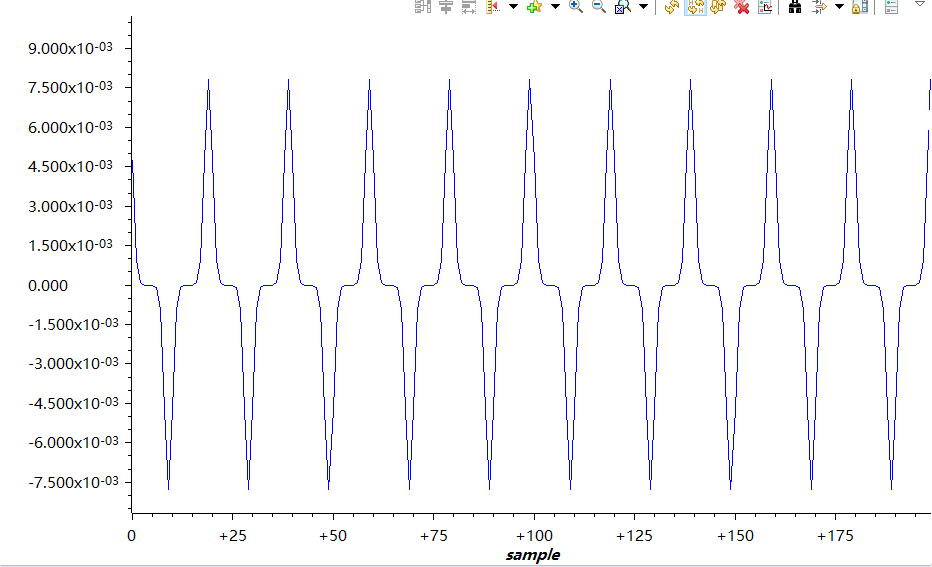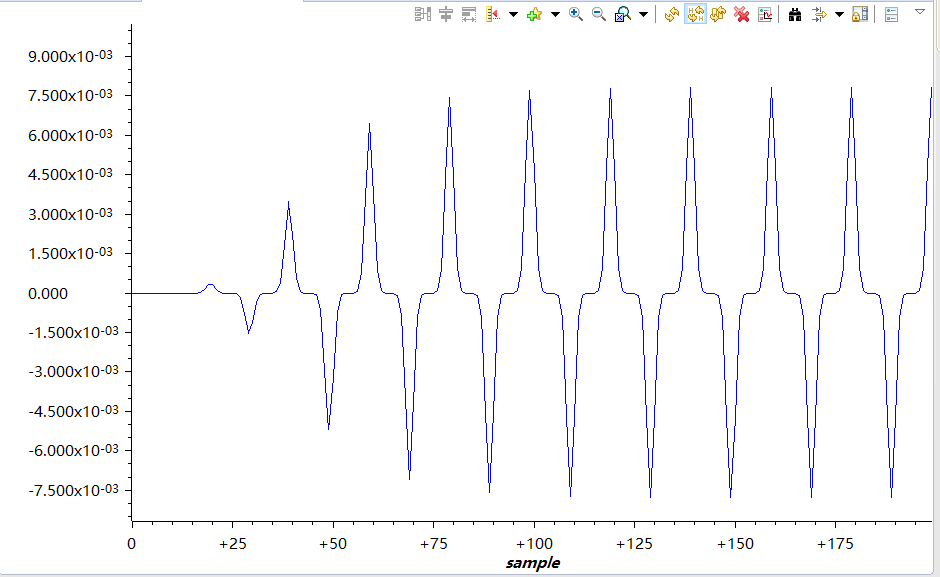08-194万+01-084012
05-28
01-241170
12-223089
05-16
04-29
10-25
07-01
12-13
05-21
09-211万+
02-106276
01-22
05-102万+
09-143万+
04-076510
09-114万+点击重新获取扫码支付余额充值